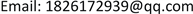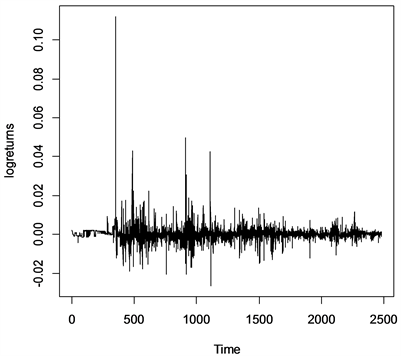﻿ 似然比扫描法在长记忆时间序列均值多变点中的应用 Application of Likelihood Ratio Scanning Method in Multiple Mean Changes in Long Memory Time Series

Hans Journal of Data Mining
Vol. 09  No. 02 ( 2019 ), Article ID: 28838 , 9 pages
10.12677/HJDM.2019.92002

Application of Likelihood Ratio Scanning Method in Multiple Mean Changes in Long Memory Time Series

Qiongyao Xu, Yuhong Xing

School of Mathematics and Statistics, Qinghai Normal University, Xining QinghaiReceived: Jan. 23rd, 2019; accepted: Feb. 6th, 2019; published: Feb. 13th, 2019ABSTRACT

Based on likelihood ratio scanning method (LRSM), this paper studies the multiple change point problem of mean in piecewise stationary long memory time series. Through numerical simulation, it is found that applying the LRSM directly to long memory time series will lead to inaccurate detection of the number and location of change points. By revising the residual estimation method of likelihood function parameters in LRSM, a new LRSM is proposed which is suitable for long memory time series. The effectiveness and practicability of the improved method are proved by numerical simulation and actual data analysis.

Keywords:Long Memory Time Series, Mean Change Points, Likelihood Ratio Scanning Statistics1. 引言

2. 似然比扫描方法

2.1. 基本设定和假设

${Y}_{t,j}={X}_{t}$ , ${\tau }_{j-1} ,

${Y}_{t,j}={\varphi }_{j0}+{\varphi }_{j1}{Y}_{t-1,j}+\cdots +{\varphi }_{j,{p}_{j}}{Y}_{t-{p}_{j},j}+{\sigma }_{j}{\epsilon }_{t}$

${\epsilon }_{t}$ 为独立同分布的白噪声序列，且均值为0，方差为1。

2.2. 用似然扫描方法估计变点的三个步骤

${W}_{t}\left(h\right)=\left\{t-h+1,\cdots ,t+h\right\}$ ,

${X}_{{W}_{t}\left(h\right)}=\left({X}_{t-h+1},\cdots ,{X}_{t+h}\right)$

$L\left(\theta \right)=\sum _{t=1}^{n}{l}_{t}\left(\theta \right)\equiv \sum _{t=1}^{n}\mathrm{log}\left\{{f}_{\theta }\left({z}_{t}/{z}_{t-1},{z}_{t-2},\cdots ,{z}_{t-p}\right)\right\}$ (1)

${S}_{h}\left(t\right)=\frac{1}{h}{L}_{1h}\left(t,{\stackrel{^}{\theta }}_{1}\right)+\frac{1}{h}{L}_{2h}\left(t,{\stackrel{^}{\theta }}_{2}\right)-\frac{1}{h}{L}_{\cdot h}\left(t,\stackrel{^}{\theta }\right)$

${S}_{h}\left(t\right)$ 统计量扫描所有的观测值可以得到一系列的似然比扫描统计量

$\left({S}_{h}\left(h\right),{S}_{h}\left(h+1\right),\cdots ,{S}_{h}\left(n-h\right)\right)$ 。如果t是变点，那么 ${S}_{h}\left(t\right)$ 的值会趋向于变大，由于选择的窗口长度为2h，

${\stackrel{^}{J}}^{\left(1\right)}=\left\{m\in \left\{h,h+1,\cdots ,n-h\right\}:{S}_{h}\left(m\right)=\underset{t\in \left(m-h,m+h\right)}{\mathrm{max}}{S}_{h}\left(t\right)\right\}$

$t 或者是 $t>n-h$ 时， ${S}_{t}\left(h\right)=0$ ，如果 ${S}_{h}\left(m\right)$ 在以点m为中心的窗口 $\left[m-h+1,m+h\right]$ 中达到最

$\text{MDL}\left(m,J,p\right)=\mathrm{log}\left(m\right)+\left(m+1\right)\mathrm{log}\left(n\right)+\sum _{j=1}^{m+1}\mathrm{log}\left({p}_{j}\right)+\sum _{j=1}^{m+1}\frac{{p}_{j}+2}{2}\mathrm{log}\left({n}_{j}\right)-\sum _{j=1}^{m+1}{L}_{j}\left({\stackrel{^}{\theta }}_{j}\right)$

$\left({\stackrel{^}{m}}^{\left(2\right)},{\stackrel{^}{J}}^{\left(2\right)},{\stackrel{^}{p}}^{\left(2\right)}\right)=\underset{\begin{array}{l}m=|J|,J\in {\stackrel{^}{J}}^{\left(1\right)}\\ p\in {\left\{1,\cdots ,{p}_{\mathrm{max}}\right\}}^{m}\end{array}}{\mathrm{arg}\mathrm{min}}\text{MDL}\left(m,J,p\right)$

${E}_{j}\left(h\right)=\left\{{\stackrel{^}{\tau }}_{j}^{\left(2\right)}-2h-1,\cdots ,{\stackrel{^}{\tau }}_{j}^{\left(2\right)}+2h\right\}$

${X}_{{E}_{j}\left(h\right)}=\left({X}_{{\stackrel{^}{\tau }}_{j}^{\left(2\right)}-2h-1},\cdots ,{X}_{{\stackrel{^}{\tau }}_{j}^{\left(2\right)}+2h}\right)$

${L}_{j}\left(\tau ,{\theta }_{1},{\theta }_{2}\right)=\sum _{t={\stackrel{^}{\tau }}_{j}^{\left(2\right)}-2h+1}^{\tau }{l}_{t}\left({\theta }_{1}\right)+\sum _{t=\tau +1}^{{\stackrel{^}{\tau }}_{j}^{\left(2\right)}+2h}{l}_{t}\left({\theta }_{2}\right)$ ，对于 $j=1,\cdots ,{\stackrel{^}{m}}^{\left(2\right)}$ ，定义最后的变点估计量为：

${\stackrel{^}{\tau }}_{j}^{\left(3\right)}=\mathrm{arg}\underset{\tau \in \left({\stackrel{^}{\tau }}_{j}^{\left(2\right)}-h,{\stackrel{^}{\tau }}_{j}^{\left(2\right)}+h\right]}{\mathrm{max}}{L}_{j}\left(\tau ,{\stackrel{^}{\theta }}_{j},{\stackrel{^}{\theta }}_{j+1}\right)$

3. 对似然比扫描方法的改进

${\left(1-L\right)}^{{d}_{0}}{X}_{t}={\epsilon }_{t}$ , $t=1,2,\cdots ,n$

4. 数值模拟Table 1. Correctly detect the number of times that there is no change pointTable 2. Correctly detect the number, position and error of one change point

Table 3. Correctly detect the number, position and error of two change points

5. 实例分析Figure 1. Yield of Shanghai composite index on January 2, 1992 solstice on December 29, 2000

6. 小结

Application of Likelihood Ratio Scanning Method in Multiple Mean Changes in Long Memory Time Series[J]. 数据挖掘, 2019, 09(02): 9-17. https://doi.org/10.12677/HJDM.2019.92002

1. 1. Page, E.S. (1955) A Test for a Change in a Parameter Occurring at an Unknown Point. Biometrika, 42, 523-527. https://doi.org/10.1093/biomet/42.3-4.523

2. 2. 王欣, 尹留志, 方兆本. 异常交易行为的甄别研究[J]. 数理统计与管理, 2009, 28(4): 671-677.

3. 3. 张丕远, 王铮, 刘啸雷. 中国近2000年气候演变的阶段性[J]. 中国科学(B), 1994, 24(9): 998-1008.

4. 4. Staudacher, M., Telser, S., Amann, A., Hinterhuber, H. and Ritsch-Marte, M. (2005) A New Method for Change Point Detection Developed for On-Line Analysis of the Heart Beat Variability during Sleep. Physica A, 349, 582-596. https://doi.org/10.1016/j.physa.2004.10.026

5. 5. Kokoszka, P. and Leipus, R. (2000) Change-Point Estimation in ARCH Models. Bernoulli, 6, 513-539. https://doi.org/10.2307/3318673

6. 6. Kokoszka, P. and Leipus, R. (1998) Change-Point in the Mean of Dependent Observations. Statistics & Probability Letters, 40, 385-393. https://doi.org/10.1016/S0167-7152(98)00145-X

7. 7. Kokoszka, P. and Leipus, R. (1999) Testing for Parameter Changes in ARCH Model. Lithuanian Mathematical Journal, 39, 182-195. https://doi.org/10.1007/BF02469283

8. 8. Kim, S., Cho, S. and Lee, S. (2000) On the CUSUM Test for Parameter Changes in GARCH(1,1) Models. Communication in Statistics-Theory and Methods, 29,445-462. https://doi.org/10.1080/03610920008832494

9. 9. Lee, S., Tokutsu, Y. and Maekawa, K. (2004) The CUSUM Test for Parameter Change in Regression Models with ARCH Error. Journal of the Japanese Statistical Society, 34, 173-188. https://doi.org/10.14490/jjss.34.173

10. 10. 马健琦, 陈占寿, 吕娜. 基于Sieve Bootstrap 方法的长记忆过程均值变点的检验[J]. 青海师范大学学报(自科版)，2017, 33(2): 34-38.

11. 11. 秦瑞兵, 田铮, 陈占寿. 独立随机序列均值多变点的非参数检验[J]. 应用概率统计, 2013, 29(5): 449-457.

12. 12. Chen, Z., Tian, Z., et al. (2012) Moving Ratio Test for Multiple Changes in Persistence. Journal of Systems Science & Complexity, 25, 582-593. https://doi.org/10.1007/s11424-012-9255-9

13. 13. Chen, Z., Jin, Z., Tian, Z., et al. (2012) Bootstrap Testing Multiple Changes in Persistence for a Heavy-Tailed Sequence. Computational Statistics & Data Analysis, 56, 2303-2316. https://doi.org/10.1016/j.csda.2012.01.011

14. 14. Yao, Y.C. (1987) Approximating the Distribution of the Maximum Likelihood Estimate of the Change-Point in a Sequence of Independent Random Variables. The Annals of Statistics, 15, 1321-1328. https://doi.org/10.1214/aos/1176350509

15. 15. Lavielle, M. and Ludena, C. (2000) The Multiple Change Points Problem for the Spectral Distribution. Bernoulii, 6, 845-869. https://doi.org/10.2307/3318759

16. 16. Davis, R.A., Lee, T.C.M. and Rodriguez-Yam, G.A. (2006) Structural Break Estimation for Non-Stationary Time Series Models. Journal of the American Statistical Association, 101, 223-239. https://doi.org/10.1198/016214505000000745

17. 17. Killick, R., Fearnhead, P. and Eckley, I.A. (2012) Optimal Detection of Change Points with a Linear Computational Cost. Journal of the American Statistical Association, 107, 1590-1598. https://doi.org/10.1080/01621459.2012.737745

18. 18. Vostrikova, L. (1981) Detecting Disorder in Multidimensional Random Processes. Soviet Mathematics, Doklady, 24, 55-59.

19. 19. Bai, J. (1997) Estimating Multiple Breaks One at a Time. Econometric Theory, 13, 315-352. https://doi.org/10.1017/S0266466600005831

20. 20. Inclan, C. and Tiao, G.C. (1994) Use of Cumulative Sums of Squares for Retrospective Detection of Change of Variance. Journal of the American Statistical Association, 89, 913-923.

21. 21. Berkes, J., Gombay, E. and Horvath, L. (2009) Testing for Changes in the Covariance Structure of Linear Processes. Journal of Statistical Planning and Inference, 139, 2044-2063. https://doi.org/10.1016/j.jspi.2008.09.004

22. 22. Fryzlewicz, P. (2014) Wild Binary Segmentation for Multiple Change-Point Detection. The Annals of Statistics, 42, 2243-2281. https://doi.org/10.1214/14-AOS1245

23. 23. Yau, C.Y. and Zhao, Z. (2016). Inference for Multiple Change Points in Time Series via Likelihood Ratio Scan Statistics. Journal of the Royal Statistical Society: Series B, 78, 895-916. https://doi.org/10.1111/rssb.12139